# Chapter 8: Vector Algebra I - Online Test

Q1. The value of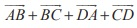is
Explaination / Solution: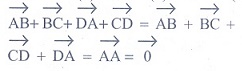Q2. Ifare parallel, then the value of m is
Explaination / Solution: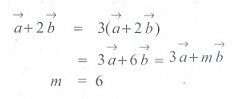Q3. The unit vector parallel to the resultant of the vectors iˆ + jˆ − kˆ and iˆ − 2 jˆ + kˆ is
Explaination / Solution:Q4. A vectormakes 60° and 45° with the positive direction of the x and y axes respectively. Then the angle betweenand the z-axis is
Explaination / Solution:Q5. If= 3iˆ + 2 jˆ + kˆ and the position vector of B is iˆ + 3jˆ − kˆ, then the position vector A is
Explaination / Solution: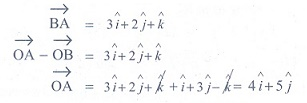Q6. A vector makes equal angle with the positive direction of the coordinate axes. Then each angle is equal to
Explaination / Solution:Q7. The vectors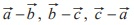are
Explaination / Solution:
No Explaination.

Q8. If ABCD is a parallelogram, thenis equal to
Explaination / Solution:Q9. One of the diagonals of parallelogram ABCD withandas adjacent sides is+. The other diagonal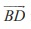is
Explaination / Solution: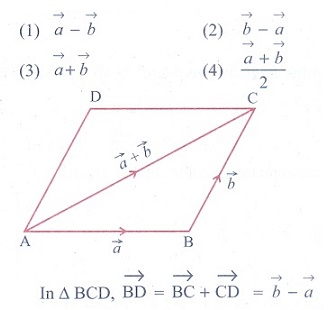Q10. If,are the position vectors A and B, then which one of the following points whose position vector lies on AB, is
Explaination / Solution: